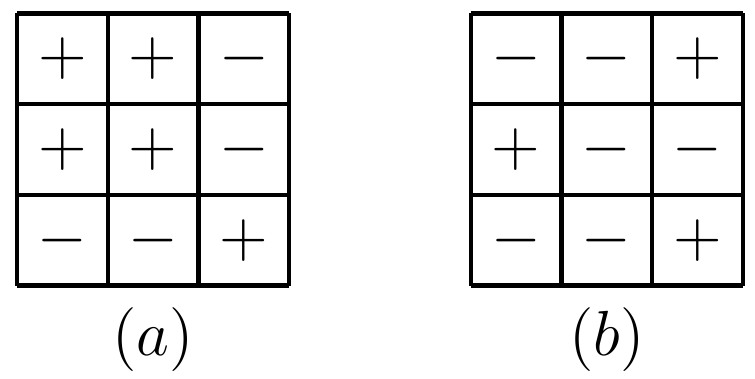###### back to index | new

Steve is piling $m \geq 1$ indistinguishable stones on the squares of an $n\times n$ grid. Each square can have an arbitrarily high pile of stones. After he finished piling his stones in some manner, he can then perform stone moves, defined as follows. Consider any four grid squares, which are corners of a rectangle, i.e. in positions $(i, k), (i, l), (j, k), (j, l)$ for some $1\leq i, j, k, l \leq n$, such that $i < j$ and $k < l$. A stone move consists of either removing one stone from each of $(i, k)$ and $(j, l)$ and moving them to $(i, l)$ and $(j, k)$ respectively,j or removing one stone from each of $(i, l)$ and $(j, k)$ and moving them to $(i, k)$ and $(j, l)$ respectively. Two ways of piling the stones are equivalent if they can be obtained from one another by a sequence of stone moves. How many different non-equivalent ways can Steve pile the stones on the grid?

$\textbf{Heaps of Beans}$

A game starts with four heaps of beans, containing $3$, $4$, $5$ and $6$ beans, respectively. The two players move alternately. A move consists of taking either one bean from a heap, provided at least two beans are left behind in that heap, or a complete heap of two or three beans. The player who takes the last bean wins. Does the first or second player have a winning strategy?

Numbers $1,2,\cdots, 1974$ are written on a board. You are allowed to replace any two of these numbers by one number which is either the sum or the difference of these numbers. Show that after 1973 times performing this operation, the only number left on the board cannot be $0$.

On an $8\times 8$ chess board, there are $32$ white pieces and $32$ black pieces, one piece in each square. If a player can change all the white pieces to black and all the black pieces to white in any row or column in a single move, then is it possible that after finitely many movies, there will be exactly one black piece left on the board?

$\textbf{Flip the Grid}$

Given two grids shown below, is it possible to transform ($a$) to ($b$) after a series of operations? In each operation, one can change all the signs in either one entire row or one entire column.$\textbf{Coin Flipping}$

There are $9$ coins on the table, all heads up. In each operation, you can flip any two of them. Is it possible to make all of them heads down after a series of operations? If yes, please list a series of such operations. If no, please explain.